Next: Conclusion Up: No Title Previous: Discussion

# Gamma-Ray Bursts

Using the obtained above dependence of compact binary merging rates in the elliptical galaxy on time (Fig.) and assuming the cosmological origin of GRB as products of binary NS/BH coalescences, we can compute the theoretical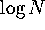-curve. To do this, we need to specify the cosmological parameters, the moment of the star formation beginning, and the spectral power-law index of a typical GRB (see Lipunov et al.  for more detail). Taking the density of baryons in stars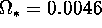(in terms of critical density to close the Universe)  and varying other parameters within limits permitted by the present theory and observations (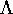-term:; the fraction of elliptical galaxies: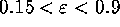; the star formation starting redshift: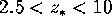), the total compact binary merging rate in the Universe (a constant the-curve goes at small fluxes) is found to vary not too much from a few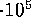yrto a few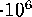yr(see Fig.).Figure: (Right panel) The 2-nd BATSE catalog (solid points) is fitted by the cosmological GRB model (from Lipunov et al. ). Note that the total GRB rate in the Universe is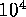per year,3 orders of magnitude smaller than the total binary NS merging rate.
Figure: (Left panel)-curves calculated for different spectral power-law indices attributable to gamma- and X-ray emission in a GRB. Values of the cosmological model parameters are shown in the Figure. The bar indicates the accessible range of the total GRB rates in the entire Universe varying the parameters as discussed in the text.

This curve is consistent with observational data obtained by BATSE (Fig.from ). However, the total GRB rate in the Universe will be of orderyr. The difference in about 3 orders can be explained either by assuming the only one merging of1000 to yield GRB, or by the gamma-ray emission collimation into a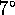solid angle .

Lipunov, Postnov, Prokhorov (1997: Astro-ph/9703181) Totany, 1997 Astro-ph/9707051) Estimate of the redshift of GRB970228 and GRB970508 using the mean statistical properties of observed GRBs. They assume the cosmological origin of GRBs as standard-candle binary neutron star mergers.Figure: Thedistribution of 3B BATSE GRBs from 256-ms 1-3 (50-300 keV) channels fitted with the cosmological model distributions in a flat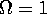Universe with a cosmological termassuming gamma-ray photon power law s=-1.1. The locations of Beppo-SAX GRBs are shown. GRB970228 and GRB970508 are marked with asterisks.Figure: The redshift - peak flux dependence in the cosmologocal models assumed for different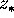and s=-1.1. 3B BATSE catalog data are also plotted.

Totany, 1997 Astro-ph/9707051

It is shown that the binary merger scenario of GRBs naturally results in the rate evolution ofup to1, that has been suggested independently from the compatibility between the number-brightness distribution and duration-brightness correlation.

Recent progress of observation of high redshift galaxies, however, gives more detailed information on the cosmic star formation history (Lilly et al. 1996; Madau et al. 1996). The Canada-France Redshift Survey (CFRS) revealed a marked evolution of 2800 Å luminosity density, that is considered to be a star formation indicator, as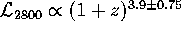to(for, ). The constant SFR approximation in spiral galaxies is therefore no longer justified even at z < 1.

The redshift of GRB970508 is likely about 2, just below the upper limit that is recently determined, and the absorption system at z = 0.835 seems not to be the site of the GRB.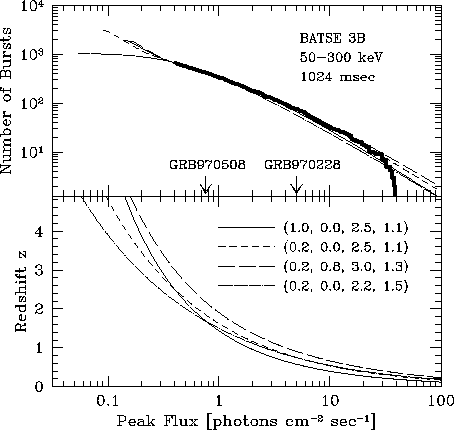Figure: The number versus peak-flux distribution (upper panel) and redshift versus peak-flux relation (lower panel). Thick solid line is the observation by BATSE (Meegan et al. 1996). Theoretical curves are calculated with SFR of the galaxy evolution model (thin-solid, long- and short-dashed lines) and with the observational SFR-evolution model (dot-dashed line). The line markings are the same for upper and lower panels. The adopted values of,,, and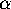are shown in the figure. The peak fluxes of GRB970228 and GRB970508 are also indicated.Next: Conclusion Up: No Title Previous: Discussion

Lipunov V.M.
Fri Nov 28 17:12:56 MSK 1997